PROBLEM #1, STATES OF MATTER

1. How much heat is needed to warm 25 grams of water from 10.ÂºC to 20.ÂºC?

(The decimals after the 10 and 20
have just been placed there to indicate that the numbers are accurate to two
significant digits.) See the graph below for a picture of what is happening in these problems.
Heat is added and the temperature of the water increases. That is all that
is happening in this problem. Leg “C” of the graph between 10.ÂºC and 20.ÂºC is the
only part of the graph that is needed. There is only a straight line graph
between these two points, meaning that this is the only thing happening and
that the entire process can be described by one equation, Q = m c Δ T.
The sloped line between the two points indicates that there is a change of heat with a change of temperature.

This problem is likely best done with W5P method because it asks for an
amount of heat when you are given two temperatures, a mass, and a type of
material. The measurements such as the specific heat, the heat of vaporization,
or the heat of fusion, the properties of those materials, are all available
if you know the type of material. In the special case of water, you should
know all of those property quantities. The English
units are not commonly used except in some industrial applications, such as
air conditioners being rated in BTU’s.

GIVEN:
T1 =10.ÂºC, T2 =20.ÂºC, m = 25 g, water is in the liquid state at the temperatures in this problem, so c = 1.00 cal/g-deg.

FIND:   a heat,
Q     The question asks for a heat in calories.

FORMULA:
Q = m c Δ T   or
Q = m c ( T2
– T1 )
You know two temperatures, a mass, and a specific heat.
You are asked to find a heat.

SOLVE:
The formula is already solved for heat as it is memorized. Substitute the known values from
GIVEN into the working equation, simplify, and do the math. No DA is
needed to change units, but carefully handle the units. The units can help find any math
problems you may have.

WHAT YOU WRITE DOWN SHOULD LOOK LIKE THIS:

GIVEN:
T1 = 10.ÂºC,
T2 = 20.ÂºC,
m = 25 g,
c= 1.00
cal/g-deg

FIND:   a heat,
QThe calculator math is:   (20 – 10) x 25 =

Calculate the numerical answer and show your cancellation of the units. The
raw answer does not need to be changed into scientific notation or shortened
to any fewer significant digits.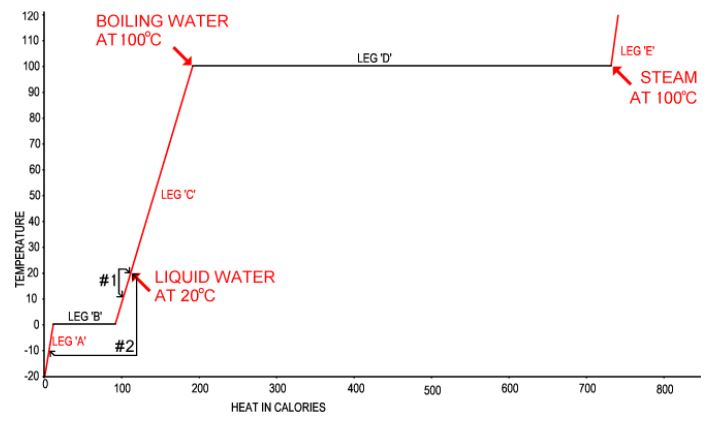PROBLEM #2, STATES OF MATTER

2. How much heat is needed to warm 25 grams of water (H
2
O) from -10.ÂºC to 20.ÂºC?

This problem involves heating ice to the melting point, a change of state (ice to water), and
heating as water. There are three legs to the temperature change of water
from -10ÂºC to 20ÂºC labelled “A” as the first leg, the warming of
ice to 0ÂºC, “B” as the melting of ice to water at 0ÂºC, and “C” as
the warming of water from 0ÂºC to 20ÂºC. The two warming stages, as
ice and as water, have the same formula, Q = m c ΔT, relating the dimensions, but the numbers
may be different. The specific heat of ice, “ci
,” is 1/2 calorie per gram-degree whereas the specific
heat of liquid water,
“cw,” is one calorie per gram degree.
The mass of the H2O is the same in this case. The
formula for the melting leg of the change is, Q = m Hf
, where Q is the heat, m is the same mass as in the other
legs, and Hf is the heat of fusion of
water. The total formula for finding the amount of heat for this temperature
and state change is the sum (total) of the three formulas as solved for Q.

The graph above shows the three legs of the change of
water from ice at -10ÂºC to 20ÂºC, and the problem solution below
labels the legs, i for ice, f for fusion, and w for water.

GIVEN:
T1i = -10ÂºC,
T2i = 0ÂºC,
m = 25 g,
ci = 1 cal/gram-degree,
T1w = 0ÂºC,
T2w = 20ÂºC,
mw = 25 g,

cw = 1 cal/gram-degree, and

Hf = 80 cal/g

FIND:   a heat, Q
The question asks for a heat in calories.

FORMULAS:
Q = m c ΔT   and   Q = m Hf

The formula for temperature increase with heat application
(Q = m c ΔT) will be used for the two legs A and
C, the increase in temperature of the H2O
as ice and as water. The formula for the energy used to melt the ice, Q = m Hf, must also be used. The total amount of heat needed must be the heat needed to warm the ice to 0 ºC, change the solid water (ice) to liquid water, still at 0 ºC, and warm the water to 20 ºC.

SOLVE:
The formulas are already solved for heat as they are memorized. The heat of
the legs must be added together to find the total heat, QT,
or, because the mass is the same in all three equations,
the equations may be rearranged as:

QT = m ci ΔTi + m Hf + m cw ΔTw
or
QT = m [(ci ΔTi) + Hf + (cw ΔTw)]

Where Q = heat in calories, c = specific heat, ΔT = the change in temperature, Hf = the heat of fusion, m = the mass, and the subscripts of “i” and “w” are for ice and water respectively. Substitute the known values from GIVEN into the working equation, simplify, and do the math.

WHAT YOU WRITE DOWN SHOULD LOOK LIKE THIS:

GIVEN:
T1i = -10ÂºC,
T2i = 0ÂºC,

m = 25 g,
ci = 1 cal/gram-degree.
T1w = 0ÂºC,
T2w = 20ÂºC,

mw = 25 g,
cw= 1 cal/gram-degree, and Hf = 80 cal/g.

FIND:   the total heat, QT

FORMULAS:
Qf = m Hf
Qi = m ci ( T2i – T1i )
Qw = m cw ( T2w– T1w)

QT = Qi + Qf + Qw

SOLVED:
QT = m ci ( T2i – T1i ) + m Hf + m cw ( T2w – T1w )

alternate:
QT = m [ci ( T2i – T1i ) + Hf + cw ( T2w – T1w )]It is fascinating that melting of ice takes a lot more energy in the form
of heat than a thirty degree change in temperature. Even so, the 80 cal/gram heat of
fusion is still a lot smaller than the 540 cal/gram heat of vaporization.

PROBLEM #3, STATES OF MATTER

3. What is the specific heat of copper metal if 200 cal increases the
temperature of 40.9 g of it from 21ÂºC to 73ÂºC?

With other temperatures, heats of phase
change, and specific heats that fit the properties of copper, the graph
for water is similar to the graph for copper metal. At the temperatures in this
problem, copper is on the first leg (Leg “A”) as a solid. There is
no change in state. The formula must be Q = m c ΔT.
The property quantities such as the value of the specific heat of copper are
to know such quantities. If you need to use such a number in your calculations and the information is not available to you in a problem, you should consult tables with these properties such as the table of heat properties of selected materials.

GIVEN:
Q = 200 cal,
T1 =
21ÂºC,
T2 =
73ÂºC,
m= 40.9 g,
The material is copper in
the solid state at the temperatures in this problem.

FIND:   a specific heat, c
The question asks for a specific heat in calories per gram.

FORMULA:  Q = m c ΔT   or   Q = m c ( T2 – T1)
You know two temperatures, a mass, and a heat.
You are asked to find a specific heat.

SOLVE:
The formula must be solved for specific heat from the memorized formula. Substitute the known values from
GIVEN into the working equation, simplify, and do the math. No DA is
needed.

WHAT YOU WRITE DOWN SHOULD LOOK LIKE THIS:

GIVEN:
Q = 200 cal,
T1 = 21ÂºC,
T2 =
73ÂºC,     m = 40.9 g,  The material is copper as a solid.

FIND:   a specific heat,
c

FORMULA:
Q = m c ΔT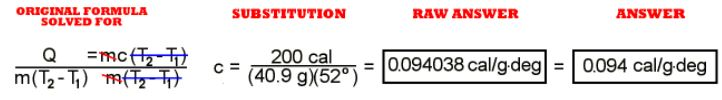The calculator math is:   200 Ã· 40.9 Ã· 52 =

Calculate the numerical answer and show your cancellation of the units. The
raw answer does not need to be changed into scientific notation but does
need to be shortened to two significant digits. How does this answer match
the value for the specific heat of copper in the table?

PROBLEM #4, STATES OF MATTER

4. How much heat is needed to increase the temperature of 12 grams of gold from 20 ÂºC to 95 ÂºC?

You know enough about the properties of gold to see that it would be a
solid in all the temperatures in this example. Q = m c ΔT is the equation that describes this change. You are given a mass,
two temperatures, and the material. You are asked for an amount of heat.
Since the type of material is available, it is assumed that you have access
to a table that would give you the specific heat,
a property of the material.

GIVEN:
T1 =
20ÂºC,
T2 =
95ÂºC,
m= 12 g,     and the material is gold in
the solid state, so from the table,
c = 0.032 cal/gram-degree.

FIND:   a heat,
Q
The question asks for a heat in calories.

FORMULA:
Q = m c ΔT   or   Q = m c ( T2 – T1
)
You know two temperatures, a mass, and a specific heat.
You are asked to find a heat. The formula comes from the formula section of Units in Lessons.

SOLVE:
The formula is already solved for heat as it is memorized. Substitute the known values from
GIVEN into the working equation, simplify, and do the math. No DA is
needed, but carefully handle the units. The units can help find any math
problems you may have.

WHAT YOU WRITE DOWN SHOULD LOOK LIKE THIS:
GIVEN:
T1 =
20ÂºC,     T2 =
95ÂºC,     m = 12 g,     c = 0.032 cal/gram-degree.

FIND:   a heat,
Q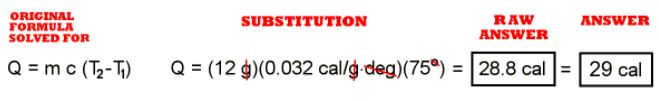The calculator math is:   12 x 0.032 x 75 =

Calculate the numerical answer and show your cancellation of the units. The
raw answer does not need to be changed into scientific notation, but it needs to be shortened
to two significant digits. It is fascinating that with only a very small
amount of added heat, the gold increases temperature so much compared to water.

PROBLEM #5, STATES OF MATTER

5. What is the temperature of 40g of water at 45ÂºC
added to 60g of water at 95ÂºC?

This is a neat little question about the
second law of thermodynamics. The
new temperature of the resulting material from a mix will all have the same
temperature and that temperature will be the weighted average of the masses
and temperatures and specific heats of the contributing materials. Since there
is no change in phase, the problem goes without that complicating factor. We
can treat the temperatures as absolute numbers, even though they are not. The
first law of thermodynamics, that energy can be traded around but not lost,
is the basis for the equation for this happening. The equations are:
Q1 + Q2
= QT  and
cT = c1
= c2  and
m1 + m2 =
mT, so
[(m1T1
) + (m2T2)
/(m1 + m2)
] = TT .

If these were different materials added together, we
would have to take into account the specific heat of each of them, but
both materials here are water in the liquid state.

For many of these problems there will be a material that gains heat
and a material that loses heat. Strictly speaking, a positive value for Q
should indicate a gain of heat (since T2
T1 is a positive number when there is
an increase in temperature) or a greater amount of the looser phase, and a
negative value for Q when there is a temperature loss or greater amount of the
more tightly – bound phase. On the other hand, if we consider “heat lost” as a
positive amount we can more easily construct formulas on the basis of,
Qgained = Qlost
rather than the slightly more inconvenient Qgained =
(Qlost), where Qlost
is a negative number.

GIVEN:
T1 =
45ÂºC
T2 =
95ÂºC
m1 = 40 g
m2 = 60 g

m1
+ m2 =
mT = 100 g         c
1
= c2 = c
T = 1.00 cal/gram-degree

FORMULAS:  Q = m c ΔT   or   Q = m c ( T2 – T1
and  Q1
+ Q2
= QT, the equation of the first law of thermodynamics, and m1
+ m2 =
the masses of water.

SOLVE:
With the several formulas, TT must be
solved for.

WHAT YOU WRITE DOWN SHOULD LOOK LIKE THIS:

GIVEN:
T1 =
45ÂºC,     T2 =
95ÂºC,     m1 = 40 g,
m2 = 60 g,

FIND:
TT, the final temperature.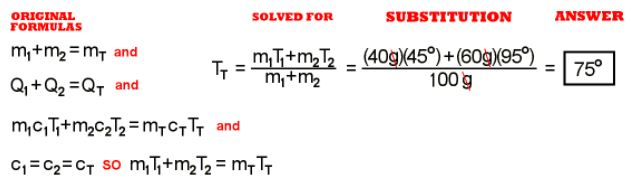The calculator math is:   40 x 45 = STO   60 x 95 + RCL = Ã· 100 =
The “STO” is a storage function and “RCL” is a reclaim function. These
may be “M+” for, “add to memory,” and “m-” for, “retrieve from memory,” or some
other such set of buttons, depending on your calculator.

Calculate the numerical answer and show your cancellation of the units. The
raw answer does not need to be changed into scientific notation or shortened
to any fewer significant digits.

PROBLEM #6, STATES OF MATTER

6. What mass of live steam at 100ÂºC is needed to heat
27.5 kg of water from 20ÂºC to 100ÂºC?

This problem is a good example of the idea that the formulas in this section
are easy, but it often takes some skill and insight to construct the set of
formulas in the correct way to reflect what is going on in the situation. See
the graph for a good mental image of what is happening in
this problem. The two materials, the steam at 100ÂºC is added to the water
at 20ÂºC. The amount of heat gained by the water to get to be water at
100ÂºC has to be the same amount of heat lost by the steam to make the same
water at 100ÂºC, the same temperature and phase as the other water.

Let’s call the amount of heat gained by the 20ÂºC water
Qc because it is a heat, the heat
on leg “c.” Similarly, let’s call the amount of heat lost by the steam
Qd because it involves leg “d.” The
formula is: Qc = Qd.
So   mc cc ΔTc =
md HV.
We know mc, cc,
ΔTc, and
HV, and we are looking for
md

WHAT YOU WRITE DOWN SHOULD LOOK LIKE THIS:

GIVEN:
T1 =
20ÂºC,
T2 =
100ÂºC,     mc = 27.5 Kg ( = 2.75 E4 g)
From the properties of water table,    cc = 1.00 cal/gram-degree,
HV = 540 cal/g

FIND:   a mass,
md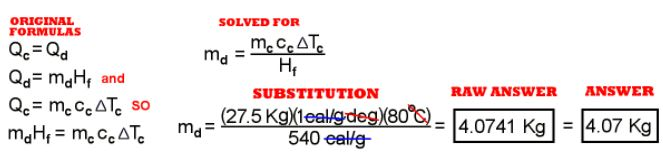The calculator math is:   27.5 X 80 Ã· 540 =

Calculate the numerical answer and show your cancellation of the units. The
raw answer does not need to be changed into scientific notation or shortened
to any fewer significant digits. It is fascinating that with only a very small
amount of added heat the gold increases temperature so much compared to water.

PROBLEM #7, STATES OF MATTER

7. A 1.1 kg (1100 gram) iron horseshoe at 1300 °C is dunked into a
bucket containing 12.8 kg of water at 20 °C. Assume that no steam was
lost. (All of the steam leaves its heat of vaporization and its mass to the
water in the bucket.) The horseshoe is taken out at 100 °C, just after
it has quit boiling the water immediately around it. What is the new temperature of the
bucket of water?

Here the horseshoe loses Q = m c ΔT heat to
the water and the water gains all that heat to increase the temperature of the
water. The first important process is to see that happening, and the second important
process is to carefully label everything so we can analyze it. Let’s call the
amount of heat lost by the iron Qi
and the amount of heat gained by the water Qw. Therefore,
Qi = Qw.

GIVEN:
T1w =
20ÂºC
T1i =
1300ÂºC
T2i = 100ÂºC
mi = 1.1 Kg
mw = 12.8 Kg
The specific heats,
ci = 0.12 cal/g-deg  and  cw = 1.00 cal/g-deg
come from the table of information on properties of materials.

FIND:
the final temperature of water, T
2w

FORMULA:
Q = m c ΔT   or   Q = m c ( T
2
– T1
)
What is more important than the memorized equations is how
to put them to use. Here for the iron horse shoe,
Qi =
mi
ci
ΔTi
or
Qi =
mi
ci
( T2i
– T1i )

and for water,
Qw =
mw
cw
ΔTw
or
Qw =
mw
cw
( T2w
– T1w )

We know the mass of iron, the specific heat of iron, the initial and final
temperatures of iron. We know the mass of water, the specific heat of water, and
the initial temperature of water. The formulas come from the formula section of Units in Chemtutor.

SOLVE:
Solve for the final temperature of the water. Substitute the known values from
GIVEN into the working equation, simplify, and do the math.

WHAT YOU WRITE DOWN SHOULD LOOK LIKE THIS:

GIVEN:
T1w =
20ÂºC,     T1i =
1300ÂºC,     T2i =
100ÂºC,     mi = 1.1 Kg,
mw = 12.8 Kg,
ci = 0.12 cal/g-deg
, and cw = 1.00 cal/g-deg.

FIND:   the
final temperature of water, T2w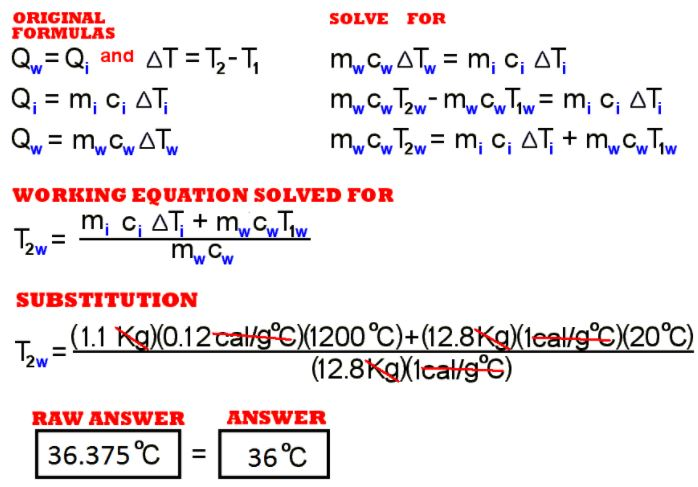The calculator math is:   1.1 x 0.12 x 1200 = STO     12.8 x 20 = + RCL = Ã· 12.8 =